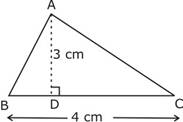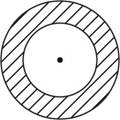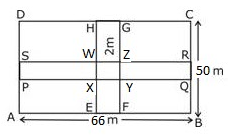### Perimeter and Area - Worksheets

CBSE Worksheet-1

CLASS –VII Mathematics (Perimeter and Area)

Choose correct option in questions 1 to 4.

1. Find the area of following triangle:a. 6 cm2
b. 5 cm2
c. 4 cm2
d. 3 cm2
2. A door frame of dimensions 4 m × 5 m is fixed on the wall of dimension 11 m × 11 m. Find the total labour charges for painting the wall if the labour charges for painting 1mof the wall is Rs 2.50.
a. Rs. 200
b. Rs. 252.50
c. Rs. 300
d. Rs. 350
3. What is the circumference of a circle of diameter 10cm?
a. 30 cm
b. 35 cm
c. 31.4 cm
d. none of these
4. Find the breadth of a rectangular plot of land, if its area is 440 mand the length is 22m.
a. 5 m
b. 10 m
c. 15 m
d. 20 m

Fill in the blanks:

1. The ______ is the distance around a given two-dimensional object.
2. If we cut a square along one of its diagonals, two triangles are obtained. Area of each triangle obtained = __________.
3. Length of rectangle = $\frac{?}{\text{Breadth of rectangle}}$
4. State true or false: All triangles equal in area are congruent.
5. A rectangular garden is 66 cm long and 50 cm wide. Two cross paths each 2 m wide are to be constructed parallel to the sides. If these paths pass through the centre of the garden, find the cost of constructing the paths at the rate Rs. 69 per m2.
6. The figure given below, shows two circles with the same centre. The radius of the larger circle is 10 cm and the radius of the smaller circle is 4 cm.
Find:
a. the area of the larger circle,
b. the area of the smaller circle,
c. the shaded area between the two circles (Take $\pi =3.14$)7. A wire is in the shape of a square of side 10 cm. If the wire is bent again into a rectangle of length 12 cm, find its breadth. Which encloses more area - the square or the rectangle?

CBSE Worksheet-1
CLASS –VII Mathematics (Perimeter and Area)

1. a
Explanation:
Area of the triangle = $\frac{1}{2}×\mathrm{b}\mathrm{a}\mathrm{s}\mathrm{e}×\mathrm{h}\mathrm{e}\mathrm{i}\mathrm{g}\mathrm{h}\mathrm{t}=\frac{1}{2}×4×3=6{\mathrm{c}\mathrm{m}}^{2}$
2. b
Explanation: Area of the wall = 11 × 11 = 121 m2
Area of the door frame = 4 × 5 = 20 m2
Area of the portion to be painted = 121 - 20 = 101 m2
Cost of painting = 101 × 2.50 = Rs. 252.50
3. c
Explanation: Circumference = $\pi$ × diameter = 3.14 × 10 = 31.4 cm
4. d
Explanation: Area of the rectangle = length × breadth = 440 m2
5. perimeter
6. $\frac{1}{4}$ × Area of the square
7. Area of rectangle
8. False
9. Rs. 15732
Explanation:Area of the path = area of the rectangle EFGH + area of the rectangle PQRS - area of the square XYZW
Area of the rectangle EFGH = 50 × 2 = 100 m2
Area of the rectangle PQRS = 66 × 2 = 132 m2
Area of the square XYZW = 2 × 2 = 4m2
Area of the path = 100 + 132 - 4 = 228 m2
Cost of constructing the path = 228 × 69 = Rs. 15732
10. a. Area of larger circle = 314cm2
b. Area of smaller circle = 50.24cm2
c. Area of shaded region = (314 - 50.24) cm= 263.76 cm2
Explanation: Area of the larger circle =  × radius2 = 3.14 × 102 = 3.14 × 100 = 314cm2
Area of the smaller circle =  × radius2 = 3.14 × 42 = 3.14 × 16 = 50.24 cm2
Area of the shaded portion = 314 - 50.224 = 263.76 cm2
11. Area of square is greater than the area of rectangle
Explanation: Since the same wire is used to make and square and rectangle, the perimeter of both the shapes will be same
Perimeter of the square = 4 × side = 4 × 10 = 40 cm
Perimeter of the rectangle = 2 ( length + breadth) = 2 (12 + breadth) = 40